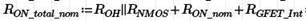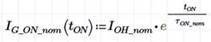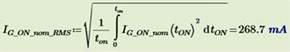If you have a related question, please click the "Ask a related question" button in the top right corner. The newly created question will be automatically linked to this question.

# UCC5350-Q1: RMS current calculation

Part Number: UCC5350-Q1

Hi Team,

My customer Preh has a question about the UCC5350-Q1:

How to calculate the RMS current for gate drivers.
4x Gate Driver UCC5530 for H-bridge control are in use and a Gate Driver will each drive an IGBT, which each IGBT 650nC gate charge has.
The switching frequency with approx. 50% duty cycle is f_sw=60kHz.
The 15V_supply supplies VCC2 at the gate driver. A transformer inductance must be dimensioned at the input of the 15V_supply and for this I need the RMS current.

1) is the RMS current calculated like this?

t_on=1/(f_sw/2)=8,3µs
I_RMS_per_driver= Q/t_on =650nC*8,3µs=78mA
I_RMS_total:= I_RMS_per_driver*2 = 156mA (2x gate drivers activated simultaneously)

2) or so?
Charge is converted as a capacitor.
C=Q/V=650nC/15V=43,3nF

Calculate Tau:=1.365ohm+3.75ohm+2.5ohm=7.6ohm
Tau_on_nom=Ron_total_nom*C=310,2ns
The current curve during capacitor charging:Peak current I_OH_nom is calculated as in the data sheet=15V/7.6ohm=1.97Ampere

Then the RMS current is calculated for the t_on duration:I_RMS_total=IG_on_nom_RMS*2 = 537mA (2x gate drivers activated simultaneously)

Thanks and best regards

David

• Hi David,

Thanks for reaching out!

Our expert is out of the office at the moment and will get back to you next week.  Thank you for your patience!

- Aaron

• David,

The method 1 should be used as it is the most accurate of average current.

Note that in general you only need to use frequency as the average current has virtually no dependency on TON, only frequency. In your case, your duty cycle is 50% so that method is correct.

Note that you have estimated the average current not necessarily RMS current.

Best

Dimitri

• Thank you